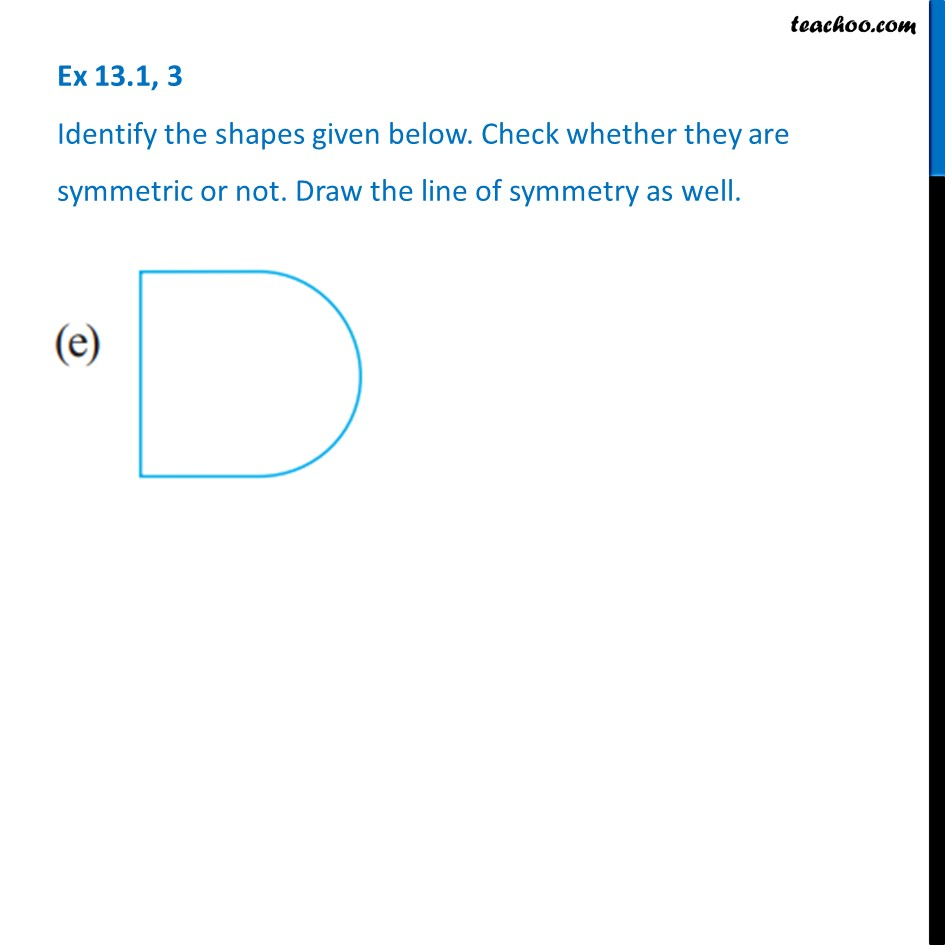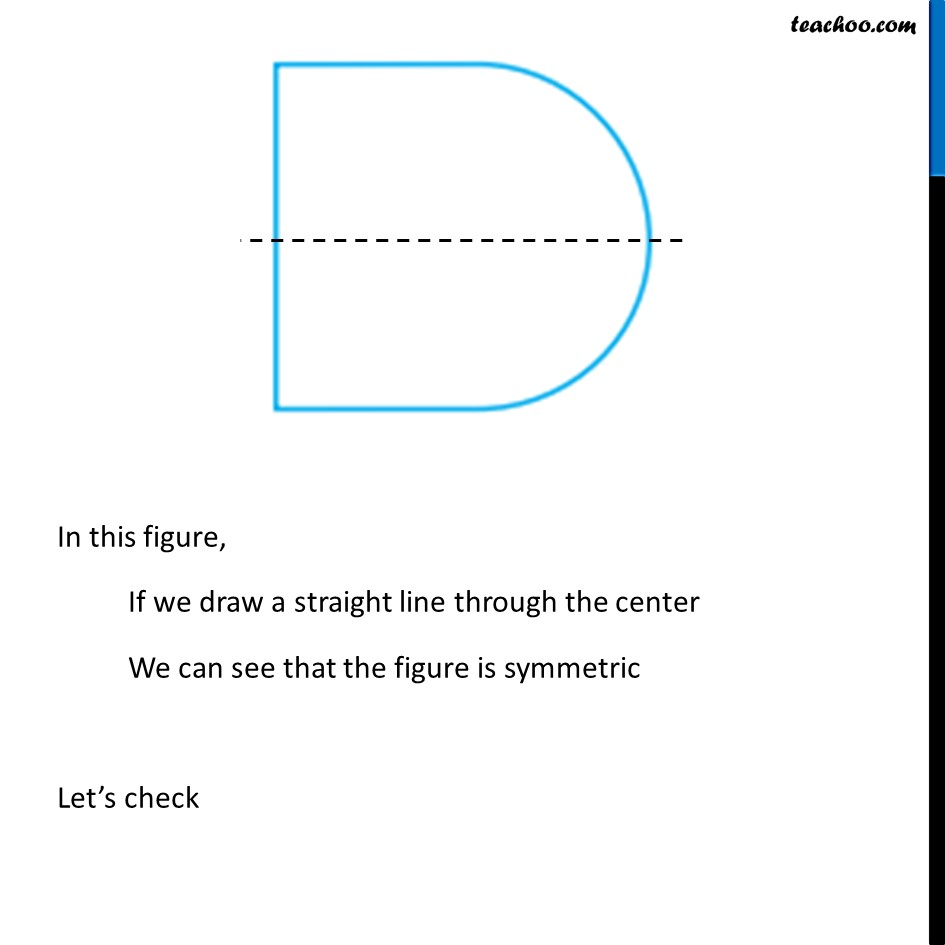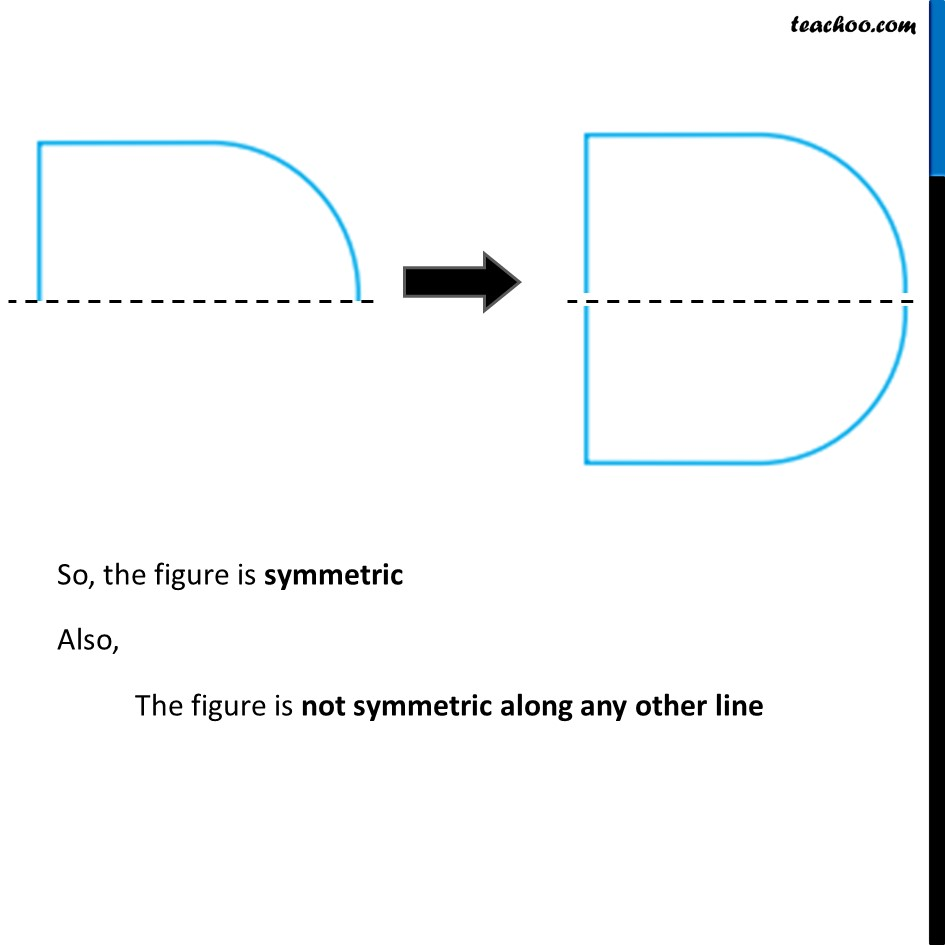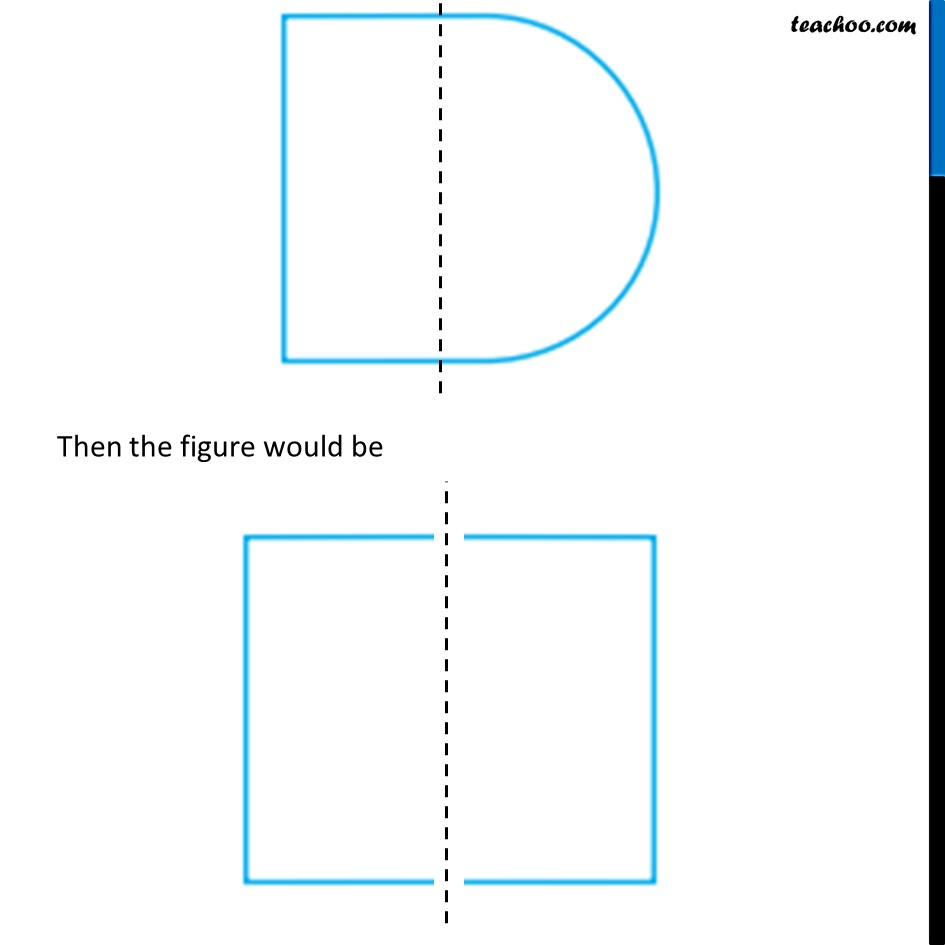Ex 13.1

Chapter 13 Class 6 Symmetry
Serial order wiseIntroducing your new favourite teacher - Teachoo Black, at only ₹83 per month

### Transcript

Ex 13.1, 3 Identify the shapes given below. Check whether they are symmetric or not. Draw the line of symmetry as well.In this figure, If we draw a straight line through the center We can see that the figure is symmetric Let’s check So, the figure is symmetric Also, The figure is not symmetric along any other line Then the figure would be